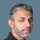cancel
Showing results for
Did you mean:Helper III

## How to use SUMPRODUCT (SUMX) in a calculated column for one related and one unrelated table? (Excel)

I have three tables and I can only relate two of them together due to circular references errors I get when trying to relate the other. I have tried to explain my goal as I've been writting this post but I think it is easier to simply show a desired output in a file.

I am looking for a formula to write in the Changes calculated column of Table 3 that gives me the desired output as shown in the worksheet. Please note this is a simplified version of my actual data and I can't change the relationship structure.

1 ACCEPTED SOLUTIONSuper User

@JollyRoger01

Add the following calculated column: I attached the file below my signature.

``````Changes =
SUMX(
FILTER(
Resource,
Resource[System] = EARLIER(System[System] )
),
Resource[Changes] *
MAXX(
FILTER(
'Weight',
'Weight'[Resource] = Resource[Resource]
),
'Weight'[Weighting]
)
)``````Did I answer your question? Mark my post as a solution! and hit thumbs up
4 REPLIES 4Super User

@JollyRoger01

Add the following calculated column: I attached the file below my signature.

``````Changes =
SUMX(
FILTER(
Resource,
Resource[System] = EARLIER(System[System] )
),
Resource[Changes] *
MAXX(
FILTER(
'Weight',
'Weight'[Resource] = Resource[Resource]
),
'Weight'[Weighting]
)
)``````Did I answer your question? Mark my post as a solution! and hit thumbs upHelper III

Worked like a charm, thank you! Out of interest, is this a common occurance and common solution?Super UserChanges Measure : =
SUMX (
RELATEDTABLE ( Changes ),
Changes[Changes] * RELATED ( Resources[Weighting] )
)

If this post helps, then please consider accepting it as the solution to help other members find it faster, and give a big thumbs up.Helper III

Thank you, however as I pointed out I cannot use a relationship to the Resources table as you have outlined. My actual relationship tree is quite complicated and it doesn't allow for a relationship to that table, so I must call it another way.